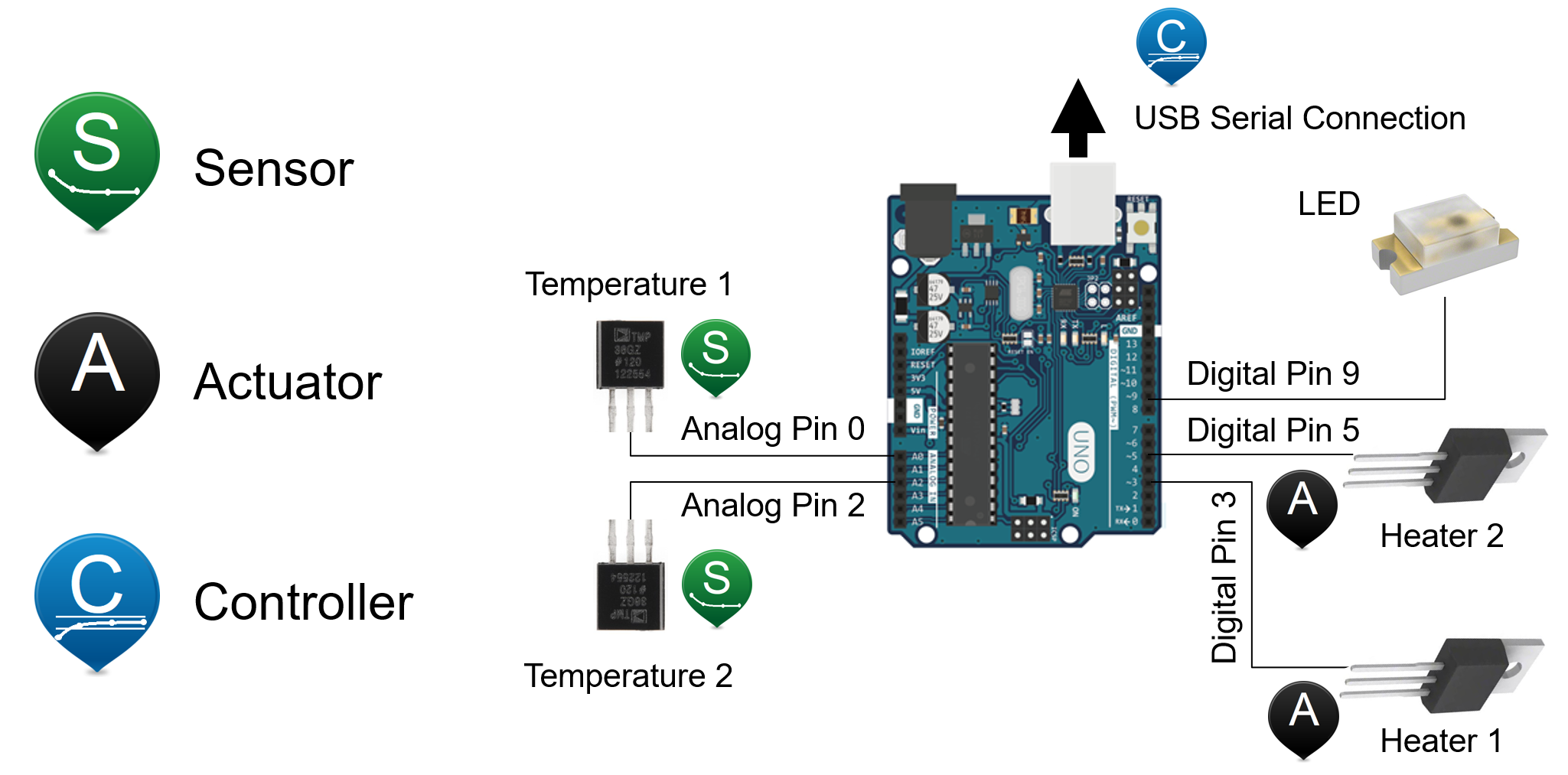Main

## TCLab Temperature Sensor

Objective: Use the TMP36 sensor correlation for converting between voltage signal and temperature.The two temperature sensors on the TCLab are TMP36GZ thermistors that report an output voltage (mV) that is linearly proportional to temperature

$$T^oC = 0.1 \, mV-50$$

TMP36 sensor accuracy is \pm 1^oC at 25^oC and \pm 2^oC over the range -40^oC to 150^oC.

• What is the gain, zero, and span of the TMP36 thermistor?
• What mV signal corresponds to 25^oC?
• What mV signal corresponds 80^oC?
• What temperature corresponds to a 0.5V signal?
• What temperature corresponds to a 1.2V signal?
• Print the current temperature for T_1 and T_2 in Celsius and milliVolts.

The mV is read on the Arduino with a 10-bit Analog to Digital Converter (ADC). There are 210 discrete levels (DL) with a 10-bit ADC between 0 and 3300 mV. The Arduino reports a DL integer between 0 and 1023. This is converted to milliVolts with mV=DL \frac{3300}{1024}. The following is a code segment from the firmware (C code) that is loaded on the Arduino.

// Arduino code tclab.ino
const int pinT1   = 0;
const int pinT2   = 2;
float mV = 0.0;
float degC = 0.0;
for (int i = 0; i < n; i++) {
mV = (float) analogRead(pinT1) * (3300.0/1024.0);
degC = degC + (mV - 500.0)/10.0;
}
degC = degC / float(n);
Serial.println(degC);

Temperature 1 (T1) is from pin 0 and temperature 2 (T2) is from pin 2 on the Arduino as shown in the instrumentation wiring schematic.The temperature is read 10 times in rapid succession and then an average is reported over the serial interface. The temperature is reported over the serial (USB) connection when Python requests a value for T1.

import time
import tclab
lab = tclab.TCLab()
print(lab.T1)
lab.close()

Solution

def T(mV):
return round(0.1*mV-50.0,1)

def mV(T):
return round((T+50.0)*10.0,2)

print('mV signal at 25 degC: ' + str(mV(25)))
print('mV signal at 80 degC: ' + str(mV(80)))
print('T at 0.5V: ' + str(T(0.5*1000)))
print('T at 1.2V: ' + str(T(1.2*1000)))

print('Current temperature for T1 and T2 in Celsius and milliVolts')
import tclab
lab = tclab.TCLab()
T1 = lab.T1
T2 = lab.T2
T1mV = (T1+50.0)*10.0
T2mV = (T2+50.0)*10.0
print('T1: '+str(T1)+' degC')
print('T2: '+str(T2)+' degC')
print('T1: '+str(round(T1mV))+' mV')
print('T2: '+str(round(T2mV))+' mV')
lab.close()